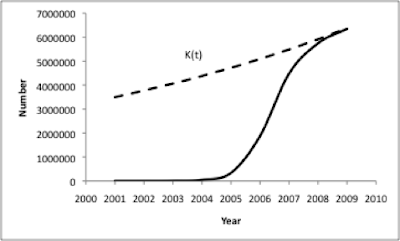## Tuesday, October 20, 2009

### Part 4 on WikiSym paper: A proposed modified model of Wikipedia Growth

As mentioned in the first post on the slowing growth rate of Wikipedia, it appears that article growth reached a peak around 2007. Rather than exponential growth, it appears that Wikipedia display logistic growth. A hypothetical logistic Lotka-Volterra population growth model bounded by a limit K is shown in the following Figure:A hypothetical logistic Lotka-Volterra population growth model bounded by a limit K.

The above figure was generated by a Lotka-Volterra population model that assumes a resource limitation K. This K variable is known as the carrying capacity, which is the limit of the population growth. Translated into our case and using the articles as the stand-in for a population, this is the maximum number of articles that Wikipedia might reach eventually. This limit might be reached because knowledge below a threshold of notability are not eligible to become an encyclopedia entry, or that there are no one around in the community who knows enough about the subject to write it up.

In either case, according to this model, at the early stages of population growth the growth rate appears exponential, but the rate decelerates as it approaches the limit K. If the total amount of encyclopedic knowledge were some constant K, then the write-up of that knowledge into Wikipedia might be expected to follow a logistic such as this above Figure.

But there is a general sense that the stock of knowledge in the world is also growing. For instance, studies of scientific knowledge (e.g., ) suggest that it exhibits exponential growth. Also, events in the world (e.g., the election of Barack Obama or Lindsey Lohan’s rehabilitations) create new possibilities for write-up.

A possible modification to the logistic growth model is as follows: We suggest that if the total amount of knowledge exhibited some monotonic growth as a function of time, K(t), one might expect a variant of logistic growth as depicted in the Figure below:A hypothetical Lotka-Volterra population growth model bound by a limit K(t) that itself grows as a function of time.

As originally recognized by Darwin in relation to the growth of biological systems , competition (the “struggle for existence”) increases as populations hit the limits of the ecology, and advantages go to members of the population that have competitive dominance over others. By analogy, we suggest that:

(a) that the population of Wikipedia editors is exhibiting a slowdown in its growth due to limited opportunities to make novel contributions, and

(b) the consequences of these (increasing) limitations in opportunities will manifest itself in increased patterns of conflict and dominance.

The limitations in opportunities might be the result of multiple and diverse constraints. For example, on one hand, we expect that the capacity parameter K is determined by limits that are internal to the Wikipedia community such as the number of available volunteers that can be coordinated together, physical hours that the editors can spend, and the level of their motivation for contributing and/or coordinating.

On the other hand, we expect that the capacity depends also on external factors such as the amount of public knowledge (available and relevant) that editors can easily forage and report on (e.g., content that are searchable on the web) and the properties of the tools that the editors and administrators are using (e.g., usability and functionalities).

In summary, globally, the number of active editors and the number of edits, both measured monthly, has stopped growing since the beginning of 2007. Moreover, the evidence suggests they follow a logistic growth function.

Our paper will finally be presented by Bongwon Suh at the WikiSym 2009 conference. The citation and link to the full paper is:
Bongwon Suh, Gregorio Convertino, Ed H. Chi, Peter Pirolli. The Singularity is Not Near: Slowing Growth of Wikipedia. In Proc. of WikiSym 2009, (to appear). Oct, 2009.

Thanks goes to my co-authors, who should receive equal credit for this research!